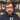# Configuring complex Luigi Pipelines

Better ways of configuring complex Luigi pipelines.by Patrick Smith

Luigi is a great tool for orchestrating complex data analysis pipelines. One potential pain point, however, is how to configure a pipeline with many tasks and, potentially, many parameters. We present a method of configuring the tasks that hopefully makes life simpler.

Consider a simple example pipeline:

``````import luigi

foo = luigi.Parameter()

def output(self):

def run(self):
with self.output().open('w') as f:
f.write('Foo is {}'.format(self.foo))

foo = luigi.Parameter()
bar = luigi.Parameter()

def requires(self):

def output(self):

def run(self):
with self.output().open('w') as f:
f.write('Bar is {}'.format(self.bar))

foo = luigi.Parameter()
bar = luigi.Parameter()

def requires(self):

def output(self):

def run(self):
with self.output().open('w') as f:
f.write('Bar is {}'.format(self.bar))

foo = luigi.Parameter()
bar = luigi.Parameter()
baz = luigi.Parameter()

def requires(self):
return {
}

def output(self):

def run(self):
with self.output().open('w') as f:
f.write('Baz is {}'.format(self.baz))``````

This defines a simple dependency graph like:

The pipeline can then be run on the command line (assuming the above code is stored in a file called `luigi_example.py`):

``luigi --module luigi_example TaskD --foo 'foo' --bar 'bar' --baz 'baz'``

One issue that you may notice is that some parameters are defined on tasks, but are just passed through to their dependencies. For example, `TaskB` does not use the `foo` parameter in its `run` method, but requires it in order to define the TaskA dependency in `requires`.

It is possible to specify the parameters only on the classes that actually use the parameters instead:

``````import luigi

foo = luigi.Parameter()

def output(self):

def run(self):
with self.output().open('w') as f:
f.write('I am {}'.format(self))

bar = luigi.Parameter()

def requires(self):

def output(self):

def run(self):
with self.output().open('w') as f:
f.write('I am {}'.format(self))

bar = luigi.Parameter()

def requires(self):

def output(self):

def run(self):
with self.output().open('w') as f:
f.write('I am {}'.format(self))

baz = luigi.Parameter()

def requires(self):
return {
}

def output(self):

def run(self):
with self.output().open('w') as f:
f.write('I am {}'.format(self))``````

However, this complicates the matter of invoking the pipeline. The command to run the pipeline is now:

``luigi --module luigi_example TaskD --baz 'baz' --TaskB-bar 'bar' --TaskC-bar 'bar' --TaskA-foo 'foo'``

## Configuration Files to the Rescue

Rather than specifying the parameter values on the command line, we can instead write a configuration file and specify that Luigi use the file to configure tasks. For example, we could use the following configuration file:

``````[TaskA]
foo: foo

bar: bar

bar: bar

baz: baz
``````

Assuming this is saved in a file `example.cfg`, we can then run the Luigi pipeline using:

``LUIGI_CONFIG_PATH=example.cfg luigi --module luigi_example TaskD``

Using a config file has the added benefit of being able to commit the file to source control to preserve the parameters used for generating a particular artifact of the Luigi pipeline.

The configuration file has a couple other tricks up its sleeve. In the above example, we’re still duplicating the value of the `bar` parameter. Since the file is parsed using `ConfigParser`, we can take advantage of the `DEFAULT` section that each task section inherits:

``````[DEFAULT]
bar: bar

foo: foo

baz: baz
``````

Note, the task must still have a section defined in the file, even though it may be empty. There’s no change to the command needed to run the pipeline with this updated file:

``LUIGI_CONFIG_PATH=example.cfg luigi --module luigi_example TaskD``

Now we have a persistent configuration that we can store is source control with no duplication of parameters and a simplified command to run it!